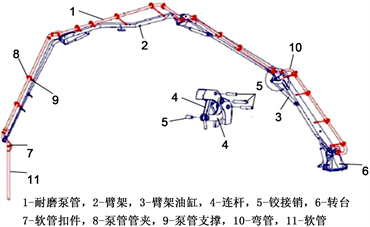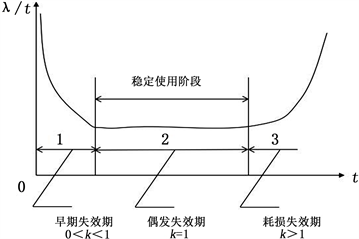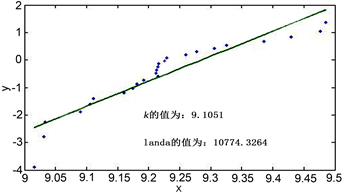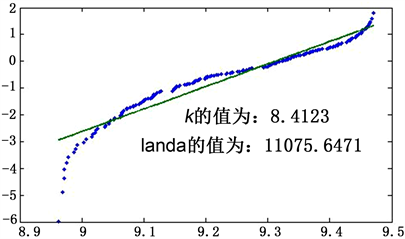# 基于威布尔分布的混凝土泵车臂架系统的剩余寿命评估Residual Life Assessment of Concrete Pump Truck Boom System Based on Weibull Distribution

• 全文下载: PDF(1402KB)    PP.443-450   DOI: 10.12677/MET.2018.76054
• 下载量: 142  浏览量: 292   科研立项经费支持

Residual life assessment of used parts of construction machinery is the premise and basis for its remanufacturing. Due to the complicated working environment of construction machinery, the traditional life prediction method is too cumbersome and complicated. In this paper, the concrete pump truck boom system is taken as an example. Based on the system component failure data and historical service condition data, the Weibull distribution and ECC system information are com-bined to evaluate the remaining life of the boom system. Improve the accuracy of the results by comparing them with the results of traditional mechanical analysis life assessments. The reliability of the actual service life and remaining life of the boom is analyzed, and the actual component reliability threshold is combined to provide a quantitative analysis basis for the remanufacturing project. It also provides a simpler method for the remanufactured residual life assessment.

1. 引言

2. 臂架系统剩余寿命评估模型Figure 1. 37 M concrete pump truck boom system structure

ECC (企业控制中心Enterprise Control Center)系统是混凝土泵车的一个定位、传感、远程传送、记录混凝土泵车运行状态及维护信息的系统。而混凝土泵车在服役过程中的运行状态和维护信息是产品面向再制造设再制造零部件寿命预测和再制造产品质量的保证。

${M}_{r}={M}_{t}-{M}_{0}$ (1)

3. 基于威布尔分布的平均寿命评估

$f\left(x\right)=\frac{k}{\lambda }{\left(\frac{x}{\lambda }\right)}^{k-1}\mathrm{exp}\left[-{\left(\frac{x}{\lambda }\right)}^{k}\right],0\le x\le \infty ,\lambda >0,k>0$ (2)

$F\left(t\right)=1-\mathrm{exp}\left[1-{\left(\frac{t}{\lambda }\right)}^{k}\right]$ (3)Figure 2. The relationship between the parameter k of the Weibull model and the failure rate

${M}_{t}=\lambda \Gamma \left(1+\frac{1}{k}\right)$ (4)

${M}_{0}=T$ (5)

${M}_{r}={M}_{t}-{M}_{0}=\lambda \Gamma \left(1+\frac{1}{k}\right)-T$ (6)

$\mathrm{ln}\left[\mathrm{ln}\left(\frac{1}{1-F\left(t\right)}\right)\right]=k\left(\mathrm{ln}t-\mathrm{ln}\lambda \right)$ (7)

$x=\mathrm{ln}t,y=\mathrm{ln}\left[\mathrm{ln}\left(\frac{1}{1-F\left(t\right)}\right)\right],A=k,B=-k\mathrm{ln}\lambda$

$\left\{\begin{array}{c}\stackrel{^}{A}=\frac{\underset{i=1}{\overset{n}{\sum }}{x}_{i}{y}_{i}-n\stackrel{¯}{x}\stackrel{¯}{y}}{\underset{i=1}{\overset{n}{\sum }}{x}_{i}^{2}-n{\stackrel{¯}{x}}^{2}}\\ \stackrel{^}{B}=\stackrel{¯}{y}-\stackrel{^}{A}\stackrel{¯}{x}\end{array}$ (8)

$F=\frac{i-0.5}{K}$ (9)

4. 实例结果对比验证Table 1. Concrete pump truck boom system failure data sheetFigure 3. Least squares fit of approximate median rank

$\lambda =10774.3264,k=9.1051$ (10)

${M}_{t}=\lambda \Gamma \left(1+\frac{1}{k}\right)=10774.3264\Gamma \left(1+\frac{1}{9.1053}\right)=10208$Figure 4. 80-bracket data sample least squares fit mapFigure 5. 200-bracket data sample least squares fit mapTable 2. 4 Typical working conditions of the boom

${M}_{t}=0.261×10336.8+0.360×12964.8+0.162×8409.6+0.216×9110.4=10687.2$

$R\left(t\right)=\mathrm{exp}\left[-{\left(\frac{t}{\lambda }\right)}^{k}\right]$ (11)

$R\left(t\right)=\mathrm{exp}\left[-{\left(\frac{9606}{10774.3264}\right)}^{9.1035}\right]=0.7308$

5. 总结

  董丽虹, 徐滨士, 董世云, 等. 金属磁记忆技术用于再制造毛坯寿命评估初探[J]. 中国表面工程, 2010, 23(2): 106-111.  Kara, S., Mazhar, M.I. and Kaebernick, H. (2004) Lifetime Prediction of Components for Reuse: An Overview. International Journal of Environmental Technology and Management, 4, 323-348. https://doi.org/10.1504/IJETM.2004.005720  Kazunori, S. and Yu, Q. (2011) Fatigue Life Evaluation Accuracy of Power Devices Using Finite Element Method. International Journal of Fatigue, 33, 1221-1234. https://doi.org/10.1016/j.ijfatigue.2011.03.009  宋守许, 张敬东, 刘志峰. 基于裂纹萌生期限的典型零件剩余寿命预测[J]. 中国机械工程, 2011, 22(20): 2490-2494.  潘权, 谢文, 贺尚红. 基于可靠性建模的混凝土泵车结构臂可再制造性评估[J]. 机械设计与研究, 2015(3): 109-113.  徐微, 胡伟明, 孙鹏. 基于两参数威布尔分布的设备可靠性预测研究[J]. 中国工程机械学报, 2013, 11(2): 112-116.  胡建军, 徐洪斌, 祖世华, 等. 服从正态分布随机载荷作用下齿轮弯曲疲劳试验研究[J]. 中国机械工程, 2013, 24(12): 1572-1575.  Shu, L.H. and Flower, W.C. (1998) Reliability Modeling in Design for Remanufacture. ASME Journal of Mechanical Design, 120, 620-627. https://doi.org/10.1115/1.2829324  Jiang, Z.H., Shu, L.H. and Benhabib, B. (2000) Reliability Analysis of Non-Constant-Size Part Population in Design for Remanufacture. ASME Journal of Mechanical Design, 122, 172-178. https://doi.org/10.1115/1.533558  方华元, 胡昌华, 黄莹, 等. 提高可靠性数据分析精度的一种有效方法[J]. 电光与控制, 2006(1): 78-80.  翁建鑫. 基于多工况分析的混凝土泵车臂架剩余疲劳寿命研究[D]: [硕士学位论文]. 湘潭: 湖南科技大学, 2014.